# DB Function

Calculates the depreciation of an asset

## What is the DB Function?

The DB function is an Excel Financial function. This function helps in calculating the depreciation of an asset. The method used for calculating depreciation is the Fixed Declining Balance Method for each period of the asset’s lifetime.

### Formula

=DB(cost, salvage, life, period, [month])

The DB function uses the following arguments:

1. Cost (required argument) – This is the initial cost of the asset.
2. Salvage (required argument) – The value of the asset at the end of the depreciation.
3. Life (required argument) – This is the useful life of the asset or the number of periods for which we will be depreciating the asset.
4. Period (required argument) – The period for which we wish to calculate the depreciation for.
5. Month (optional argument) – Specifies how many months of the year are used in the calculation of the first period of depreciation. If omitted, the function will take the default value of 12.

### How to use the DB Function in Excel?

To understand the uses of the DB function, let’s consider a few examples:

#### Example 1

Assume we wish to calculate the depreciation for an asset with an initial cost of \$100,000. The asset’s salvage value after 5 years is \$10,000.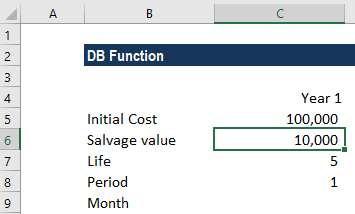We will calculate the depreciation for all five years. The formula used is: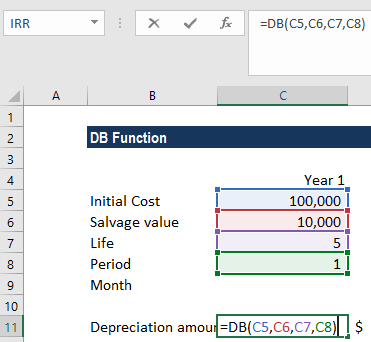The month argument was left blank, so the function would assume it as 12. For each year, we will only change the period, as shown below: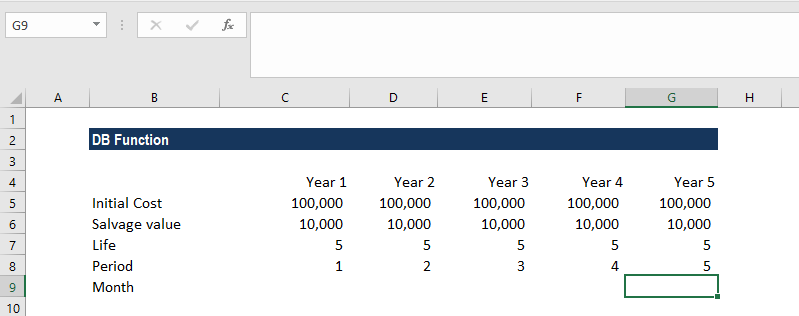The result we got for five years is below: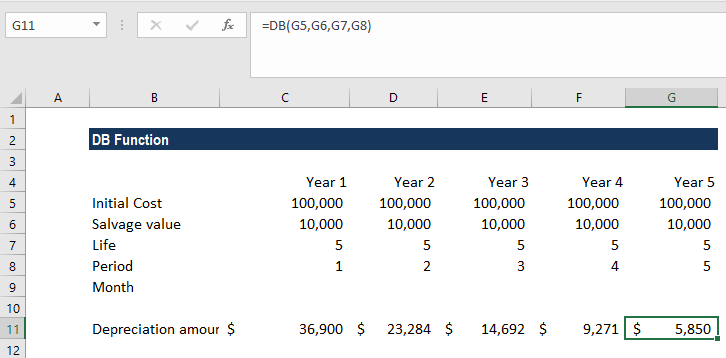The DB function uses the fixed-declining balance method to compute the asset’s depreciation at a fixed rate. The formula used by DB to calculate depreciation for a period is:

=(Cost – Total depreciation from prior periods) * Rate

Where:

Rate = 1 – ((salvage / cost) ^ (1 / life)).

Different formulas are used for first and last periods. For the first period, DB uses the following formula:

=Cost * Rate * Month / 12

For the last period, DB uses the following formula:

=((Cost – Total depreciation from prior periods) * Rate * (12 – month)) / 12

#### Example 2

Let’s take another example to understand the function more. We use the same data as in the example above. However, the depreciation calculation starts 6 months into Year 1. The data used for calculating the depreciation is: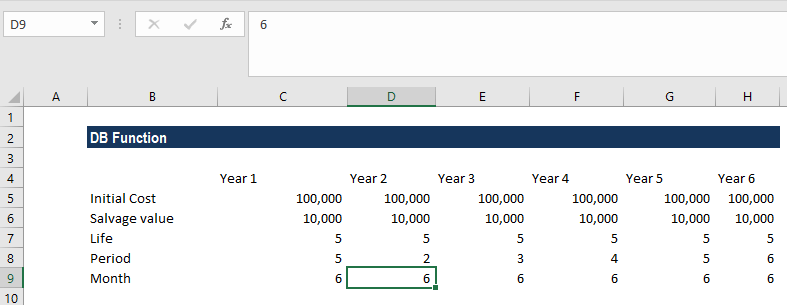Now, the new formula will make a reference to the month argument. The formula used is: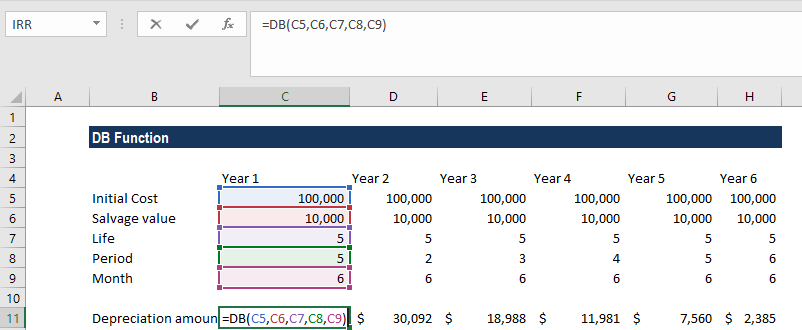In the example, the value at the end of Year 1 has only depreciated over 6 months and the value at the end of Year 2 has depreciated over 18 months (6 months of Year 1 + 12 months of Year 2), etc.

We get the result below: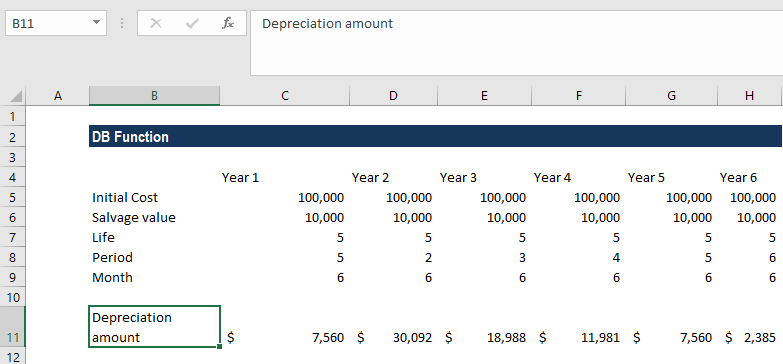### Things to remember about the DB Function

1. #VALUE! error – Occurs when the given arguments are non-numeric.
2. #NUM! error – Occurs when:
• The given cost or salvage argument is less than zero.
• The given life or period argument is less than or equal to zero.
• The given month argument is less than or equal to zero or is greater than 12.
• The given period argument is greater than the life argument and we omitted the month argument.
• The given period is greater than life+1. For example, if the life of the asset is 5 years and we provided the period argument as 6 years with 12 months as the month argument.

Thanks for reading CFI’s guide to the Excel DB function. By taking the time to learn and master Excel functions, you’ll significantly speed up your financial analysis. To learn more, check out these additional CFI resources:

• Excel Functions for Finance